’╗┐

### Introduction

#### Abstract

En
In this paper, some basic properties (recurrence relations,asymptotic expansion, series representation and others) are derived for the new function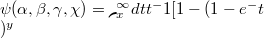$\psi(\alpha,\beta,\gamma,\chi) = \lmoustache _{x}^ \infty {dt t^\alppha-1 [1-(1- {{e^-t} \frac {t^\beta}})^y}$, which covers certain well-known special functions for particular choices of the parameteres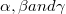$\alpha, \beta and \gamma$. For example, for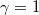$\gamma= 1$ and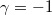$\gamma = -1$,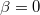$\beta = 0$ one obtains respectively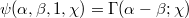$\psi(\alpha,\beta,1,\chi) = \Gamma(\alpha - \beta; \chi)$ and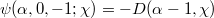$\psi(\alpha,0,-1;\chi) = -D(\alpha - 1,\chi)$,where(Error rendering LaTeX formula) is the complementary incomplete Gamma function and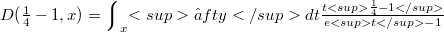$D(­Øø╝ - 1,x) = \Bigl\lmoustache _{x}Ōłłfty dt {{t­Øø╝ - 1} \over {et - 1}}$ is a function of Debye type.The function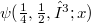$\psi(­Øø╝,­ØøĮ,╬│;x)$ has been introduce by us in order to find a class of exact solutions for nonlinear wave equations of the Klein-Gordon type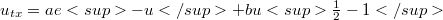$u_{tx} = ae-u + bu­ØøĮ - 1$, where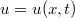$u = u(x,t)$ and a, b are constants.

DOI Code: ¦

Full Text: PDFThis work is licensed under a Creative Commons Attribuzione - Non commerciale - Non opere derivate 3.0 Italia License.